# Creating Spirals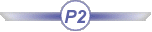This task shows how to create curves in the shape of spirals, that is a in 2D plane, as opposed to the helical curves.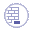Open the Spiral1.CATPart document.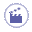1. Click Spiral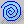.

 The Spiral Curve Definition dialog box appears.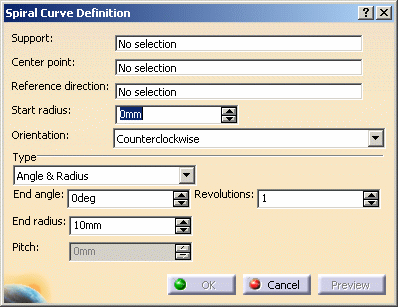2. Select a supporting plane and the Center point for the spiral.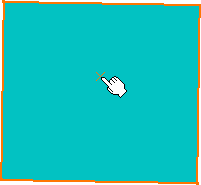3. Specify a Reference direction along which the Start radius value is measured and from which the angle is computed, when the spiral is defined by an angle.

 The spiral is previewed with the current options: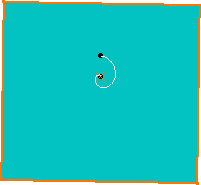4. Specify the Start radius value, that is the distance from the Center point, along the Reference direction, at which the spiral's first revolution starts.

5. Define the spiral's Orientation, that is the rotation direction: clockwise or counter clockwise.

6. Specify the spiral creation mode, and fill in the corresponding values:

• Angle & Radius: the spiral is defined by a given End angle from the Reference direction and the radius value, the radius being comprised between the Start and End radius, on the first and last revolutions respectively (i.e. the last revolution ends on a point which distance from the center point is the End radius value).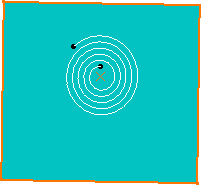Ref. direction = Z, Start radius = 5mm, Angle = 45°,  End radius = 20mm, Revolutions = 5
• Angle & Pitch: the spiral is defined by a given End angle from the Reference direction and the pitch, that is the distance between two revolutions of the spiral.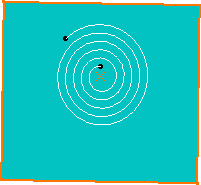• Radius & Pitch: the spiral is defined by the End radius value and the pitch. The spiral ends when the distance from the center point to the spiral's last point equals the End radius value. Ref. direction = Z, Start radius = 5mm,  End radius = 20mm, Pitch = 4mm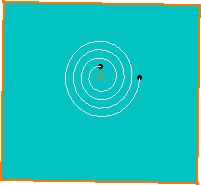Ref. direction = Z, Start radius = 5mm, Angle = 45°,  Pitch = 4mm, Revolutions = 5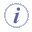Depending on the selected creation mode, the End angle, End radius, Pitch, and  Revolutions fields are available or not.
7. Click OK to create the spiral curve.

 The curve (identified as Spiral.xxx) is added to the specification tree.Parameters can be edited in the 3D geometry. To have further information, refer to the Editing Parameters chapter.